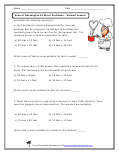Home > Topics >

## Area and Perimeter WorksheetsWe work on finding the area of many simple figures. We later advance to using our critical thinking skills to find the area of odd shaped figures. Students usually find that area problems very challeneging and perimeter problems are a bit more easy.

Area of Rectangles In Word Problems

Area of Squares Word Problems

Area of Triangle Using Trigonometry

Finding the Area of Odd Shapes

Perimeter of Polygons In Word Problems

Recognize Area Word Problems

Similar Polygons: Ratio of Perimeters & Areas

Simple Area of a Rectangle

The Area of Rectangles

Using Tiling to Find Area